## Direct Sum (Module)

The direct sum of two Modules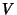and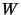over the same Ring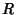is given by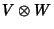with Module operations defined by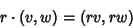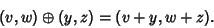The direct sum of an arbitrary family of Modules over the same Ring is also defined. If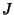is the indexing set for the family of Modules, then the direct sum is represented by the collection of functions with finite support fromto the union of all these Modules such that the function sends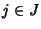to an element in the Module indexed by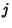.

The dimension of a direct sum is the product of the dimensions of the quantities summed. The significant property of the direct sum is that it is the coproduct in the category of Modules. This general definition gives as a consequence the definition of the direct sum of Abelian Groups (since they are Modules over the Integers) and the direct sum of Vector Spaces (since they are Modules over a Field).